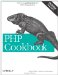# Recipe 2.5. Generating Random Numbers Within a Range

#### 2.5.1. Problem

You want to generate a random number within a range of numbers.

#### 2.5.2. Solution

Use mt_rand( ):

`// random number between \$upper and \$lower, inclusive \$random_number = mt_rand(\$lower, \$upper);`

#### 2.5.3. Discussion

Generating random numbers is useful when you want to display a random image on a page, randomize the starting position of a game, select a random record from a database, or generate a unique session identifier.

To generate a random number between two endpoints, pass mt_rand( ) two arguments:

`\$random_number = mt_rand(1, 100);`

Calling mt_rand( ) without any arguments returns a number between 0 and the maximum random number, which is returned by mt_getrandmax( ) .

Generating truly random numbers is hard for computers to do. Computers excel at following instructions methodically; they're not so good at spontaneity. If you want to instruct a computer to return random numbers, you need to give it a specific set of repeatable commands; the fact that they're repeatable undermines the desired randomness.

PHP has two different random number generators, a classic function called rand( ) and a better function called mt_rand( ). MT stands for Mersenne Twister , which is named for the French monk and mathematician Marin Mersenne and the type of prime numbers he's associated with. The algorithm is based on these prime numbers. Since mt_rand( ) is less predictable and faster than rand( ), we prefer it to rand( ).

If you're running a version of PHP earlier than 4.2, before using mt_rand( ) (or rand( )) for the first time in a script, you need to seed the generator by calling mt_srand( ) (or srand( )). The seed is a number the random function uses as the basis for generating the random numbers it returns; it's used to solve the repeatable versus random dilemma mentioned earlier. Use the value returned by microtime( ), a high-precision time function, to get a seed that changes very quickly and is unlikely to repeat'qualities desirable in a good seed. After the initial seed, you don't need to reseed the randomizer. PHP 4.2 and later automatically handle seeding for you, but if you manually provide a seed before calling mt_rand( ) for the first time, PHP doesn't alter it by substituting a new seed of its own.

If you want to select a random record from a database , an easy way is to find the total number of fields inside the table, select a random number in that range, and then request that row from the database, as in Example 2-1.

##### Selecting a random row from a database

 `query('SELECT COUNT(*) AS count FROM quotes'); if (\$row = \$sth->fetchRow()) { \$count = \$row; } else { die (\$row->getMessage()); } \$random = mt_rand(0, \$count - 1); \$sth = \$dbh->query("SELECT quote FROM quotes LIMIT \$random,1"); while (\$row = \$sth->fetchRow()) { print \$row . "\n"; } ?>`

This snippet finds the total number of rows in the table, computes a random number inside that range, and then uses LIMIT \$random,1 to SELECT one line from the table starting at position \$random.

Alternatively, if you're using MySQL 3.23 or above, you can do this:

`\$sth = \$dbh->query('SELECT quote FROM quotes ORDER BY RAND() LIMIT 1'); while (\$row = \$sth->fetchRow()) {     print \$row . "\n"; }`

In this case, MySQL randomizes the lines, and then the first row is returned.

Recipe 2.6 for how to generate biased random numbers; documentation on mt_rand( ) at http://www.php.net/mt-rand and rand( ) at http://www.php.net/rand; the MySQL Manual on rand( ) is found at http://www.mysql.com/doc/M/a/Mathematical_functions.html.PHP Cookbook: Solutions and Examples for PHP Programmers
ISBN: 0596101015
EAN: 2147483647
Year: 2006
Pages: 445

Similar book on Amazon